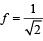Courses

# Test: Gravitation 2 - From Past 28 Years Questions

## 30 Questions MCQ Test Physics 28 Years Past year papers for NEET/AIPMT Class 11 | Test: Gravitation 2 - From Past 28 Years Questions

Description
This mock test of Test: Gravitation 2 - From Past 28 Years Questions for NEET helps you for every NEET entrance exam. This contains 30 Multiple Choice Questions for NEET Test: Gravitation 2 - From Past 28 Years Questions (mcq) to study with solutions a complete question bank. The solved questions answers in this Test: Gravitation 2 - From Past 28 Years Questions quiz give you a good mix of easy questions and tough questions. NEET students definitely take this Test: Gravitation 2 - From Past 28 Years Questions exercise for a better result in the exam. You can find other Test: Gravitation 2 - From Past 28 Years Questions extra questions, long questions & short questions for NEET on EduRev as well by searching above.
QUESTION: 1

### The distance of two planets from the sun are 1013 and 1012 metres respectively. The ratio of time periods of these two planets is 

Solution:

T2 ∝ R3 (Kepler's law)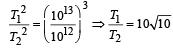QUESTION: 2

### The largest and the shortest distance of the earth from the sun are r1 and r2. Its distance from the sun when it is at perpendicular to the major-axis of the orbit drawn from the sun is 

Solution:

Applying the properties of ellipse, we have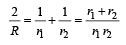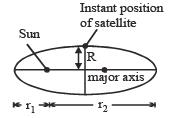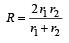QUESTION: 3

### If the gravitational force between two objects were proportional to 1/R (and not as 1/R2) where R is separation between them, then a particle in circular orbit under such a force would have its orbital speed v proportional to 

Solution: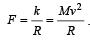Hence v ∝ R0

QUESTION: 4

For a satellite escape velocity is 11 km/s. If the satellite is launched at an angle of 60° with the vertical, then escape velocity will be 

Solution:

Since, escape velocity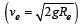is independent of angle of projection, so it will not change.

QUESTION: 5

A planet is moving in an elliptical orbit around the sun. If T, V, E and L stand respectively for its kinetic energy, gravitational potential energy, total energy and magnitude of angular momentum about the centre of force, which of the following is correct ? 

Solution:

In a circular or elliptical orbital motion, torque is always acting parallel to displacement or velocity. So, angular momentum is conserved.
In attractive field, potential energy is negative.
Kinetic energy changes as velocity increase when distance is less. So, option (c) is correct.

QUESTION: 6

A satellite of mass m is orbiting around the earth in a circular orbit with a velocity v. What will be its total energy? 

Solution:

Total energy =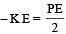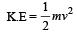QUESTION: 7

A seconds pendulum is mounted in a rocket. Its period of oscillation decreases when the rocket 

Solution: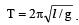. When the rocket accelerates upwards g increases to ( g + a).

QUESTION: 8

The mean radius of earth is R, its angular speed on its own axis is ω and the acceleration due to gravity at earth's surface is g. What will be the radius of the orbit of a geostationary satellite ? 

Solution: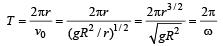Hence,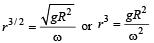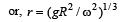QUESTION: 9

A satellite A of mass m is at a distance of r from the surface of the earth. Another satellite B of mass 2m is at a distance of 2r from  the earth’s centre. Their time periods are in the ratio of 

Solution: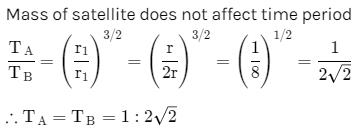QUESTION: 10

The escape velocity from earth is 11.2 km/s. If a body is to be projected in a direction making an angle 45° to the vertical, then the escape velocity is

Solution:

Escape velocity does not depend on the angle of projection.

QUESTION: 11

The distance of Neptune and Saturn from the sun is nearly 1013 and 1012 meter respectively.Assuming that they move in circular orbits, their periodic times will be in the ratio 

Solution:

T2 ∝ R3 (According to Kepler ’s law)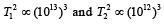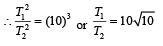QUESTION: 12

A satellite in force free space sweeps stationary interplanetary dust at a rate dM/dt = αv where M is the mass and v is the velocity of the satellite and α is a constant. What is the deceleration of the satellite? 

Solution: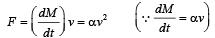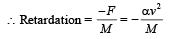QUESTION: 13

The escape velocity from the surface of the earth is ve.The escape velocity from the surface of a planet whose mass and radius are three times those of the earth, will be 

Solution:

Escape velocity on surface of earth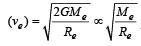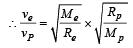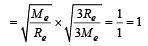or,   vP= ve.

QUESTION: 14

What will be the formula of the mass in terms of g, R and G (R = radius of earth) 

Solution:

We know that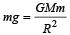QUESTION: 15

A ball is dropped from a satellite revolving around the earth at a height of 120 km. The ball will 

Solution:

The orbital speed of satellite is independent of mass of satellite, so the ball will behave as a satellite and will continue to move with the same speed in the original orbit.

QUESTION: 16

The period of revolution of planet A around the Sun is 8 times that of B. The distance of A from the Sun is how many times greater than that of B from the Sun? 

Solution:

(a) : Period of revolution of planet   A(TA)=8TB
According to Kepler's III law of planetary motion
T2∝R3

Therefore;

=> (rA / rB)= (TA / TB)2

=> (8TA / TB)2 = 64

=> rA / rB = 4

or  r= 4 rB

QUESTION: 17

The escape velocity of a body on the surface of the earth is 11.2 km/s. If the earth’s mass increases to twice its present value and the radius of the earth becomes half, the escape velocity would become 

Solution:

Escape velcocity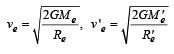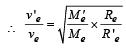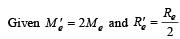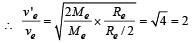v 'e = 2ve = 2 x 11.2 = 22.4 km/s

QUESTION: 18

The escape velocity of a sphere of mass m is given by (G = Universal gravitational constant; M = Mass of the earth and Re = Radius of the earth)     

Solution:

Escape velocity is the minimum velocity with which a body is projected to escape from earth's gravitational field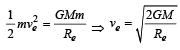QUESTION: 19

The escape velocity on the surface of earth is 11.2 km/s. What would be the escape velocity on the surface of another planet of the same mass but 1/4 times the radius of the earth? 

Solution: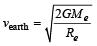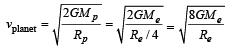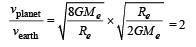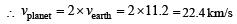QUESTION: 20

A body weighs 72 N on the surface of the earth.What is the gravitational force on it due to earth at a height equal to half the radius of the earth from the surface? 

Solution:

mg = 72 N  (body weight on the surface)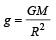At a height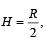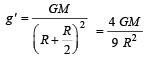Body weight at height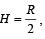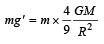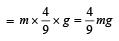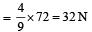QUESTION: 21

Assuming earth to be a sphere of uniform density, what is the value of ‘g’ in a mine 100 km below the earth’s surface? (Given, R = 6400 km) 

Solution:

We know that effective gravity g' at depth below earth surface is given by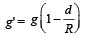Here, d = 100 km, R = 6400 km,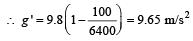QUESTION: 22

The potential energy of a satellite, having mass m and rotating at a height of 6.4 × 106m from the earth surface, is 

Solution:

Mass of the satellite = m and heigh t of satellite from earth (h) = 6.4 × 106 m.
We know that gravitational potential energy of the satellite at height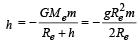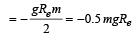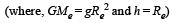QUESTION: 23

With what velocity should a particle be projected so that its height becomes equal to radius of earth?           

Solution:

From conservation of energy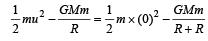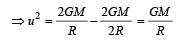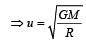QUESTION: 24

Assuming the radius of the earth as R, the change in gravitational potential energy of a body of mass m, when it is taken from the earth's surface to a height 3R above its surface, is 

Solution:

Gravitational potential energy (GPE) on the surface of earth,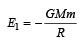GPE at 3R,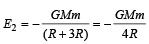∴ Change in GPE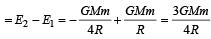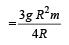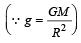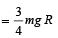QUESTION: 25

The acceleration due to gravity on the planet A is 9 times the acceleration due to gravity on planet B. A man jumps to a height of 2m on the surface of A. What is the height of jump by the same person on the planet B? 

Solution:

Applying conservation of total mechanical energy principle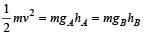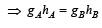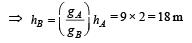QUESTION: 26

Two spheres of masses m and M are situated in air and the gravitational force between them is F.The space around the masses is now filled with a liquid of specific gravity 3. The gravitational force will now be 

Solution:

Gravitational force is independent of medium, Hence, this will remain same.

QUESTION: 27

The density of a newly discovered planet is twice that of earth. The acceleration due to gravity at the surface of the planet is equal to that at the surface of the earth. If the radius of the earth is R, the radius of the planet would be

Solution: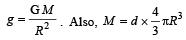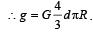At the surface of planet,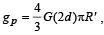At the surface of the earth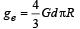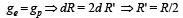QUESTION: 28

Imagine a new planet having the same density as that of earth but it is 3 times bigger than the earth in size. If the acceleration due to gravity on the surface of earth is g and that on the surface of the new planet is g’, then 

Solution:

We know that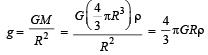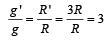∴  g ' = 3g

QUESTION: 29

For a satellite moving in an or bit ar ound the earth, the ratio of kinetic energy to potential energy is 

Solution:

K.E. of satellite moving in an orbit around the earth  is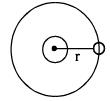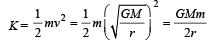P.E. of satellite and earth system is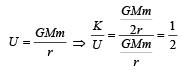QUESTION: 30

The Earth is assumed to be a sphere of radius R.
A platform is arranged at a height R from the surface of the Earth. The escape velocity of a body from this platform is fv, where v is its escape velocity from the surface of the Earth.
The value of f is 

Solution:

Potential energy at height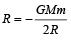If m be the mass of a body which is thrown with velocity ve so that it goes out of gravitational field from distance R, then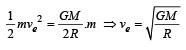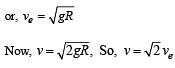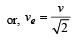Comparing it with given equation,